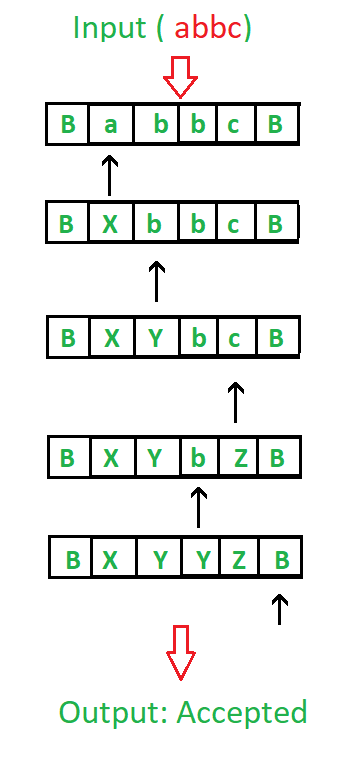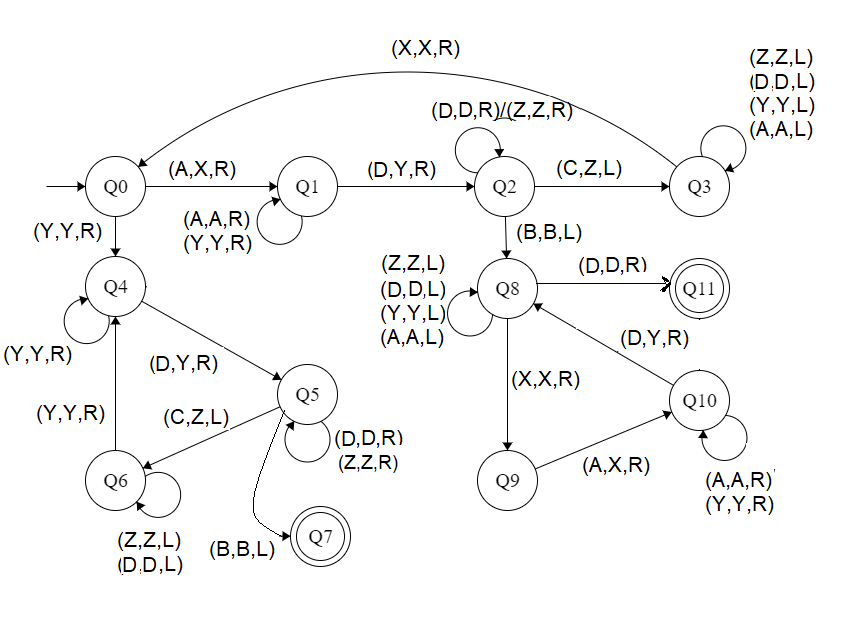Construct a Turing Machine for a language L = {aibjck | i<j<k or i>j>k} ∩ {aibjck | i>j>k or i>j>k}

Prerequisite – Turing Machine
The language L = {aibjck | i < j < k or i > j > k} ∩ {aibjck | i > j > k or i > j > k} is same as the languages L={aibjck | j > max(i, k)}
In this language, every string of ‘a’, ‘b’ and ‘c’ have certain number of a’s, then certain number of b’s and then certain number of c’s.

The condition is as follows

• Count of 1st symbols should be atleast 1. ‘b’ and ‘c’ can have thereafter be as many but count of a is less than count of ‘b’ and count of ‘b’ is less than count of ‘c’.
• And, The count of 3rd symbols should be atleast 1. ‘a’ and ‘b’ can have thereafter be as many but count of c is less than count of ‘b’ and count of ‘b’ is less than count of ‘a’
• On Conclusion: Count of 2nd symbol must be greater than max of count of 1st and 3rd symbol and count of 1st and 3rd symbol may or may not be equal
• Assume that string is ending with ‘\$’.

Examples:

Input: a a a b b c
Here a = 3, b = 2, c = 1
Output: NOT ACCEPTED
// |a| < |b| > |c|

Input: a b b b c c
Here a = 1, b = 3, c = 2
Output: ACCEPTED

Input: a a a b b b b c c c
Here a = 3, b = 4, c = 3
Output: ACCEPTED

Tape Representation:Approach:

1. Camparing two elements by making two element as a single element.
2. If |Second| is greater than max(|First|, |Third|), then it is accepted.
3. Else it is not accepted.

Steps:

• Step-1: Convert A into X and move right and goto step 2. If Y is found ignore it and move right to step-5.
• Step-2: Keep ignoring A and Y and move towards right. Convert D into Y and move right and goto step-3.
• Step-3: Keep ignoring D and Z and move towards right. If C is found make it Z and move left to step 4.If B is found ignore it and move left and goto step-8.
• Step-4: Keep ignoring Z, A, Y and D and move towards left. If X is found ignore it and move right and goto step-1.
• Step-5: Keep ignoring Y and move towards right. If D is found make it Y and move right to step-6.
• Step-6: Keep ignoring D and Z and move towards right. Convert C into Z and move left and goto step-7. If B Ignore it move left and goto step-11.
• Step-7: Keep ignoring D and Z and move towards left. If Y is found ignore it and move right and goto step-5.
• Step-8: Keep ignoring D, Y and A and move towards left. Ignore X move right and goto step-9. If D ignore and move right and goto step-11
• Step-9: Convert A into X and move right and goto step-10.
• Step-10: Keep ignoring Y and A and move towards right. If D make it Y and move right and goto step-8.
• Step-11: Stop the Machine (String is accepted).

State transition diagram :Here, Q0 shows the initial state and Q1, Q2, Q3, Q4, Q5, Q6, Q8, Q9, Q10 shows the transition state and Q7 and Q11 shows the final state. A, C, D are the variables used and R, L shows right and left.

Explanation:

• Using Q0, when A is found make it X and go to right and to state Q1.And, when Y is found ignore it and go to right and to state Q4
• On the state Q1, ignore all A and Y and goto right. If D found make it Y and goto right into next state Q2.
• In Q2, ignore all D, Z and move right. If B found ignore it, move left and goto the state Q4, If C found make it Z move left and to Q3.
• In Q3 state, ignore all Z, D, Y, A and move left. If X found ignore it move right to Q0.
• In Q4, ignore all Y and move right. If D is found make it Y and move to right to Q5.
• In Q5 state, ignore all D, Z and move right. If C found make it Z move left to state Q6.If B is found ignore move left to state Q7
• In Q6, ignore all D, Z and move left. If Y found ignore it and move right to state Q4.
• If Q7 state is reached it will produced the result of acceptance of string.
• In Q8, ignore all A, Y, D and move left. If X found ignore it move right to state Q9. If B is found ignore it and move right to Q11.
• In Q9 state, if A found make it X move right to state Q10
• In Q10, ignore all A, Y and move right. If D found make it Y and move right to state Q8.
• If Q11 state is reached it will produced the result of acceptance of string.

Note: For comparison of |A|, |D|, |C|, the concept of Turing Machine as Comparator is usedMy Personal Notes arrow_drop_upSmall things always make you to think big

If you like GeeksforGeeks and would like to contribute, you can also write an article using contribute.geeksforgeeks.org or mail your article to contribute@geeksforgeeks.org. See your article appearing on the GeeksforGeeks main page and help other Geeks.

Please Improve this article if you find anything incorrect by clicking on the "Improve Article" button below.

Article Tags :

Be the First to upvote.

Please write to us at contribute@geeksforgeeks.org to report any issue with the above content.